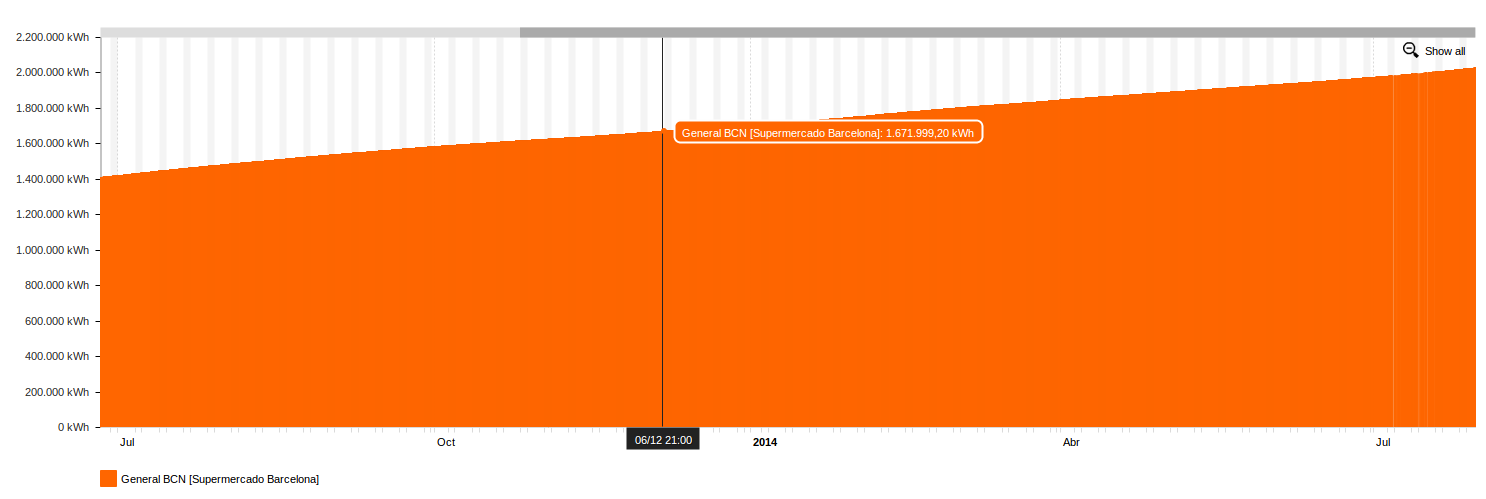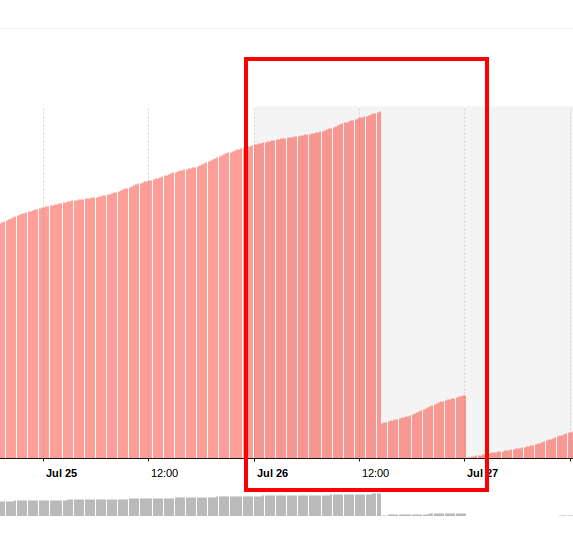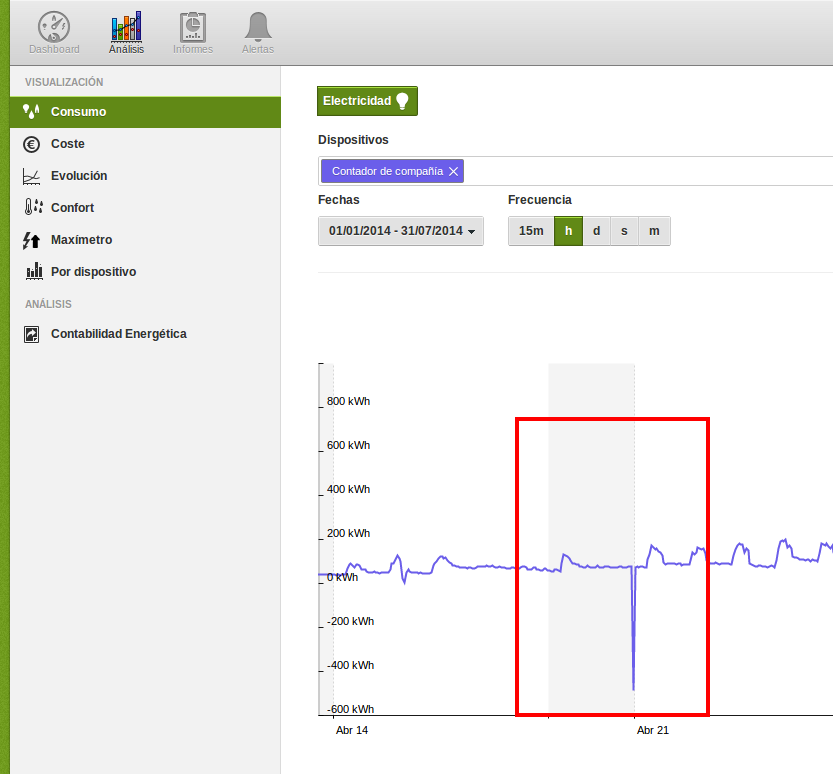# Understanding Active Energy in the platform

The different kind of meters you have installed in your facility could send data discretely or cumulatively.

Usually, Energy data will be sent in a cumulated way. DEXCell Energy Manager can work with both values, but it should be clear what represents each type of parameters.

Let's put an example of a fiscal meter. The facility will have an energy meter which will cumulate a numerical value according to the consumed kWh. This value indicates what the fiscal meter has consumed from the beginning. In order to know how much energy we have consumed on a specific time interval (such as 15 min), we should make the difference between two consecutive quarters of an hour.

So, if Active Energy values are the following:

• 01/01/2018 at 15:00 --> 422283,16 kWh
• 01/01/2018 at 15:15 --> 422309,47 kWh

That means that in this specific 15min, we have consumed 26,31 kWh (which is the difference between both quarters). So, from 15:00 to 15:15 we have consumed 26,31 kWh.

That is the reason why we need to understand that these values are aggregated incrementally. Otherwise, in the calculation of the discrete value, we would obtain negative values or peaks.Sometimes, the al meter will download quarter-hourly data in a daily way (for example, calling to the fiscal meter daily), so each set of data will start from 0.## Import historical data from Excel

If we want to import historical data or fill some gaps of data in the case our datalogger had a problem and couldn't send the data, we should keep in mind how we are going to import these data.

• If we are going to import cumulated Active Energy data, we must import it using the 402 parameters. DEXCell Energy Manager will calculate automatically the discrete parameters (15min, h, d, s and m)
• In the case we want to import directly the discrete parameters, we must indicate the sampling frequency. That is, if our readings are quarter hourly, we have to use the 40201 parameter. If our data is hourly, we will have to use the 40211 parameter. And so on. For more information about any other frequency, click here.

## Common mistakes

• Import discrete data using the 402 parameter. This will make that DEXCell Energy Manager calculate the quarter hourly differences and the obtained values will be negatives. because it expected cumulated data.
• Import Active Energy data (cumulated) into a discrete parameter, such as 40201. This will show on analysis menus cumulated energy, and not the discrete one, which is what we have to work on.
• Import data without taking into account the previous cumulated data into the platform. This will make appear a positive or negative peak.